#### 期刊菜单

Riemann-Liouville型分数阶扩散方程的显–隐和隐–显差分方法及数值分析
Explicit-Implicit and Implicit-Explicit Difference Methods and Numerical Analysis for Riemann-Liouville Type Fractional Diffusion Equation

Abstract: For the Riemann-Liouville (R-L) type fractional differential equations describing sub-diffusion phenomena, a kind of explicit-implicit and implicit-explicit difference schemes for numerically solving this problem is constructed. It takes advantage of the fast computation of explicit formats and the unconditional stability of implicit formats, alternatingly applies the classical explicit format and the implicit format by time layer. Then, using the Fourier method analysis, the format is unconditionally stable and convergent. The numerical test results are consistent with the theoret-ical analysis results, and show that the computational efficiency of the explicit-implicit and implic-it-explicit formats is better than the classical implicit format. The explicit-implicit and implic-it-explicit methods are feasible to solve the R-L fractional diffusion equation.

1. 引言

$\frac{\partial u\left(x,t\right)}{\partial t}={}_{0}D{}_{t}^{1-\gamma }\left[\frac{{\partial }^{2}u\left(x,t\right)}{\partial {x}^{2}}\right]+f\left(x,t\right),\text{}t\ge 0$ . (1)

${}_{0}D{}_{t}^{1-\gamma }u\left(x,t\right)=\frac{1}{\Gamma \left(\gamma \right)}\frac{\partial }{\partial t}{\int }_{0}^{t}\frac{u\left(x,\tau \right)}{{\left(t-\tau \right)}^{1-\gamma }}\text{d}\tau$ .

2. 分数阶扩散方程显–隐和隐–显方法

2.1. R-L型时间分数阶扩散方程

$\frac{\partial u\left(x,t\right)}{\partial t}={}_{0}D{}_{t}^{1-\gamma }\left[\frac{{\partial }^{2}u\left(x,t\right)}{\partial {x}^{2}}\right]+f\left(x,t\right),\text{\hspace{0.17em}}\text{\hspace{0.17em}}0 . (2)

${}_{0}D{}_{t}^{\gamma -1}\left(\frac{\partial u\left(x,t\right)}{\partial t}\right)={}_{0}D{}_{t}^{\gamma -1}\left\{{}_{0}D{}_{t}^{1-\gamma }\left[{K}_{\gamma }\frac{{\partial }^{2}u\left(x,t\right)}{\partial {x}^{2}}\right]+f\left(x,t\right)\right\}$ ,(3)

${}_{0}{}^{C}D{}_{t}^{\gamma }\frac{\partial u\left(x,t\right)}{\partial t}={K}_{\gamma }\frac{{\partial }^{2}u\left(x,t\right)}{\partial {x}^{2}}-{\left\{{}_{0}D{}_{t}^{-\gamma }\left[{K}_{\gamma }\frac{{\partial }^{2}u\left(x,t\right)}{\partial {x}^{2}}\right]\right\}|}_{t=0}+{}_{0}D{}_{t}^{\gamma -1}f\left(x,t\right)$ , (4)

${\left\{{}_{0}D{}_{t}^{-\gamma }\left[{K}_{\gamma }\frac{{\partial }^{2}u\left(x,t\right)}{\partial {x}^{2}}\right]\right\}|}_{t=0}=0$ .

${}_{0}^{C}{D}_{t}^{\gamma }\frac{\partial u\left(x,t\right)}{\partial t}={K}_{\gamma }\frac{{\partial }^{2}u\left(x,t\right)}{\partial {x}^{2}}+g\left(x,t\right),g\left(x,t\right)={}_{0}D{}_{t}^{\gamma -1}f\left(x,t\right)$ (5)

2.2. 显–隐和隐–显格式的构造

${t}_{k}=k\tau ,k=0,1,\cdots ,N$${x}_{j}=jh,j=0,1,\cdots ,M$$\tau =T/N$$h=L/M$。定义网格函数

${u}_{i}^{n}=u\left({x}_{i},{t}_{n}\right)$ , ${f}_{i}^{n}=f\left({x}_{i},{t}_{n}\right)$ , ${g}_{i}^{n}=g\left({x}_{i},{t}_{n}\right)$ , $\left({x}_{i},{t}_{n}\right)\in \left[0,L\right]×\left[0,T\right]$ .

${D}_{\tau }^{\gamma }{w}^{n}=\frac{{\tau }^{-\gamma }}{\Gamma \left(2-\gamma \right)}\left[{w}^{n}-\underset{k=1}{\overset{n-1}{\sum }}\left({a}_{n-k-1}-{a}_{n-k}\right){w}^{k}-{a}_{n-1}{w}^{0}\right]$ , ${a}_{k}={\left(k+1\right)}^{1-\alpha }-{k}^{1-\alpha }$ .

$\begin{array}{l}\frac{1}{\Gamma \left(1-\alpha \right)}{\int }_{0}^{{t}_{n}}\frac{{y}^{\prime }\left(\xi \right)\text{d}\xi }{{\left(t-\xi \right)}^{\alpha }}-\frac{{\tau }^{-\alpha }}{\Gamma \left(2-\alpha \right)}\left[{y}^{n}-\underset{k=1}{\overset{n-1}{\sum }}\left({a}_{n-k-1}-{a}_{n-k}\right){y}^{k}-{a}_{n-1}{y}^{0}\right]\\ \le \frac{1}{\Gamma \left(2-\alpha \right)}\left[\frac{1-\alpha }{12}+\frac{{2}^{2-\alpha }}{2-\alpha }-\left(1+{2}^{\alpha }\right)\right]\underset{0\le t\le {t}_{n}}{\mathrm{max}}|{y}^{″}\left(t\right)|{\tau }^{2-\alpha }\end{array}$ .

$\frac{{\tau }^{-\gamma }}{\Gamma \left(2-\gamma \right)}\left[{u}_{j}^{k}-\underset{i=1}{\overset{k-1}{\sum }}\left({a}_{k-i-1}-{a}_{k-i}\right){u}_{j}^{i}-{a}_{k-1}{u}_{j}^{0}\right]={\delta }_{x}^{2}{u}_{j}^{k}+{g}_{j}^{k}$ ,(6)

$\frac{{\tau }^{-\gamma }}{\Gamma \left(2-\gamma \right)}\left[{u}_{j}^{k}-\underset{i=1}{\overset{k-1}{\sum }}\left({a}_{k-i-1}-{a}_{k-i}\right){u}_{j}^{i}-{a}_{k-1}{u}_{j}^{0}\right]={\delta }_{x}^{2}{u}_{j}^{k-1}+{g}_{j}^{k},\text{\hspace{0.17em}}\text{\hspace{0.17em}}j=1,2,\cdots ,M-1$ . (7)

$\begin{array}{l}{u}_{0}^{k}=\phi \left({t}_{k}\right),\text{\hspace{0.17em}}\text{\hspace{0.17em}}{u}_{m}^{k}=\psi \left({t}_{k}\right),\text{\hspace{0.17em}}\text{\hspace{0.17em}}k=0,1,\cdots ,N\\ {u}_{j}^{0}=w\left({x}_{j}\right),\text{\hspace{0.17em}}\text{\hspace{0.17em}}j=0,1,\cdots ,M\end{array}$

$\left\{\begin{array}{l}\frac{{\tau }^{-\gamma }}{\Gamma \left(2-\gamma \right)}\left[{u}_{j}^{2k+1}-\underset{i=1}{\overset{2k}{\sum }}\left({a}_{2k-i}-{a}_{2k-i+1}\right){u}_{j}^{i}-{a}_{2k}{u}_{j}^{0}\right]={\delta }_{x}^{2}{u}_{j}^{2k}+{g}_{j}^{2k+1},\\ \frac{{\tau }^{-\gamma }}{\Gamma \left(2-\gamma \right)}\left[{u}_{j}^{2k+2}-\underset{i=1}{\overset{2k+1}{\sum }}\left({a}_{2k-i+1}-{a}_{2k-i}\right){u}_{j}^{i}-{a}_{2k+1}{u}_{j}^{0}\right]={\delta }_{x}^{2}{u}_{j}^{2k+2}+{g}_{j}^{2k+2},\end{array}$ (8)

$\left\{\begin{array}{l}\frac{{\tau }^{-\gamma }}{\Gamma \left(2-\gamma \right)}\left[{u}_{j}^{2k+1}-\underset{i=1}{\overset{2k}{\sum }}\left({a}_{2k-i}-{a}_{2k-i+1}\right){u}_{j}^{i}-{a}_{2k}{u}_{j}^{0}\right]={\delta }_{x}^{2}{u}_{j}^{2k+1}+{g}_{j}^{2k+1},\\ \frac{{\tau }^{-\gamma }}{\Gamma \left(2-\gamma \right)}\left[{u}_{j}^{2k+2}-\underset{i=1}{\overset{2k+1}{\sum }}\left({a}_{2k-i+1}-{a}_{2k-i}\right){u}_{j}^{i}-{a}_{2k+1}{u}_{j}^{0}\right]={\delta }_{x}^{2}{u}_{j}^{2k+1}+{g}_{j}^{2k+2}.\end{array}$ (9)

3. 显–隐和隐–显方法的稳定性分析

$\left\{\begin{array}{l}{u}_{j}^{2k+1}=\left({a}_{0}-{a}_{1}-2\mu \right){u}_{j}^{2k}+\mu {u}_{j+1}^{2k}+\mu {u}_{j-1}^{2k}+\underset{i=1}{\overset{2k-1}{\sum }}\left({a}_{2k-i}-{a}_{2k-i+1}\right){u}_{j}^{i}-{a}_{2k}{u}_{j}^{0}+\mu {g}_{j}^{2k+1},\\ \left(1+2\mu \right){u}_{j}^{2k+2}-\mu {u}_{j+1}^{2k+2}-\mu {u}_{j-1}^{2k+2}=\left({a}_{0}-{a}_{1}\right){u}_{j}^{2k+1}+\underset{i=1}{\overset{2k}{\sum }}\left({a}_{2k-i+1}-{a}_{2k-i+2}\right){u}_{j}^{i}-{a}_{2k+1}{u}_{j}^{0}+\mu {g}_{j}^{2k+2},\end{array}$ (10)

${U}_{j}^{k}$ 是显–隐格式的近似解，定义 ${\rho }_{j}^{k}={u}_{j}^{k}-{U}_{j}^{k}$ $\left(k=1,2,\cdots ,N,j=1,2,\cdots ,M-1\right)$${\rho }^{k}={\left[{\rho }_{1}^{k},{\rho }_{2}^{k},\cdots ,{\rho }_{M-1}^{k}\right]}^{\text{T}}$，代入(10)式可得如下误差扰动方程：

$\left\{\begin{array}{l}{\rho }_{j}^{2k+1}=\left({a}_{0}-{a}_{1}-2\mu \right){\rho }_{j}^{2k}+\mu {\rho }_{j+1}^{2k}+\mu {\rho }_{j-1}^{2k}+\underset{i=1}{\overset{2k-1}{\sum }}\left({a}_{2k-i}-{a}_{2k-i+1}\right){\rho }_{j}^{i}-{a}_{2k}{\rho }_{j}^{0},\\ \left(1+2\mu \right){\rho }_{j}^{2k+2}-\mu {\rho }_{j+1}^{2k+2}-\mu {\rho }_{j-1}^{2k+2}=\left({a}_{0}-{a}_{1}\right){\rho }_{j}^{2k+1}+\underset{i=1}{\overset{2k}{\sum }}\left({a}_{2k-i+1}-{a}_{2k-i+2}\right){\rho }_{j}^{i}-{a}_{2k+1}{\rho }_{j}^{0},\end{array}$ (11)

${\rho }^{k}\left(x\right)=\left\{\begin{array}{l}0,\text{}0\le x\le \frac{h}{2}\text{,}\\ {\rho }_{j}^{k},\text{}{x}_{j}-\frac{h}{2} $\left(k=1,2,\cdots ,N\right)$

${\rho }^{k}\left(x\right)$ 的Fourier展开形式为：

${\rho }^{k}\left(x\right)=\underset{l=-\infty }{\overset{\infty }{\sum }}{d}_{k}\left(l\right){\text{e}}^{i2\pi lx/L}$ ,

$\begin{array}{c}{‖{\rho }^{k}‖}_{2}^{2}=\underset{j=1}{\overset{M-1}{\sum }}h{|{\rho }_{j}^{k}|}^{2}\\ ={\int }_{0}^{\frac{h}{2}}{|{\rho }^{k}\left(x\right)|}^{2}\text{d}x+\underset{j=1}{\overset{M-1}{\sum }}{\int }_{{x}_{j}-\frac{h}{2}}^{{x}_{j}+\frac{h}{2}}{|{\rho }^{k}\left(x\right)|}^{2}\text{d}x+{\int }_{L-\frac{h}{2}}^{L}{|{\rho }^{k}\left(x\right)|}^{2}\text{d}x\\ ={\int }_{0}^{L}{|{\rho }^{k}\left(x\right)|}^{2}\text{d}x\end{array}$ (12)

${\int }_{0}^{L}{|{\rho }^{k}\left(x\right)|}^{2}\text{d}x=L\underset{m=-\infty }{\overset{\infty }{\sum }}{|{d}_{k}\left(m\right)|}^{2}$，即 ${‖{\rho }^{k}‖}_{2}^{2}=L\underset{m=-\infty }{\overset{\infty }{\sum }}{|{d}_{k}\left(m\right)|}^{2}$ .

$m=1$ 时(m是时间层数)，我们使用显式差分格式，有

${\rho }_{j}^{1}=\left(1-2\mu \right){\rho }_{j}^{0}+\mu {\rho }_{j+1}^{0}+\mu {\rho }_{j-1}^{0}$ ,

${d}_{1}=\left(\mu {\text{e}}^{i\sigma h}+1-2\mu +\mu {\text{e}}^{-i\sigma h}\right){d}_{0}$ ,

${\text{e}}^{±i\theta }=\mathrm{cos}\theta ±i\mathrm{sin}\theta$，可得

$|{d}_{1}|=|1-4\mu {\mathrm{sin}}^{2}\frac{\sigma h}{2}||{d}_{0}|\le C|{d}_{0}|$ , (13)

$m=2$ 时，第一层使用显式格式，第二层使用隐式格式，

${\rho }_{j}^{1}=\left(1-2\mu \right){\rho }_{j}^{0}+\mu {\rho }_{j+1}^{0}+\mu {\rho }_{j-1}^{0}$ ,

$\left(1+2\mu \right){\rho }_{j}^{2}-\mu {\rho }_{j+1}^{2}-\mu {\rho }_{j-1}^{2}=\left({a}_{0}-{a}_{1}\right){\rho }_{j}^{1}+{a}_{1}{\rho }_{j}^{0}$ ,

$\left(1+4\mu {\mathrm{sin}}^{2}\frac{\sigma h}{2}\right){d}_{2}=\left({a}_{0}-{a}_{1}\right)\left(1-4\mu {\mathrm{sin}}^{2}\frac{\sigma h}{2}\right){d}_{0}+{a}_{1}{d}_{0}$ ,

$|{d}_{2}|=|\frac{\left(1-{a}_{1}\right)\left(1-4\mu {\mathrm{sin}}^{2}\frac{\sigma h}{2}\right)+{a}_{1}}{1+4\mu {\mathrm{sin}}^{2}\frac{\sigma h}{2}}||{d}_{0}|\le |\frac{1-\left(1-{a}_{1}\right)4\mu {\mathrm{sin}}^{2}\frac{\sigma h}{2}}{1+4\mu {\mathrm{sin}}^{2}\frac{\sigma h}{2}}||{d}_{0}|\le C|{d}_{0}|$ .(14)

$m=2k$ 时，假设 $|{d}_{n}|\le C|{d}_{0}|,n\le 2k$ 成立；当 $m=2k+2$，有

$\left\{\begin{array}{l}{d}_{2k+1}=\left({a}_{0}-{a}_{1}-4\mu {\mathrm{sin}}^{2}\frac{\sigma h}{2}\right){d}_{2k}+\underset{i=1}{\overset{2k-1}{\sum }}\left({a}_{2k-i}-{a}_{2k-i+1}\right){d}_{i}-{a}_{2k}{d}_{0},\\ \left(1+4\mu {\mathrm{sin}}^{2}\frac{\sigma h}{2}\right){d}_{2k+2}=\left({a}_{0}-{a}_{1}\right){d}_{2k+1}+\underset{i=1}{\overset{2k}{\sum }}\left({a}_{2k-i+1}-{a}_{2k-i+2}\right){d}_{j}-{a}_{2k+1}{d}_{0},\end{array}$

$\begin{array}{l}\left(1+4\mu {\mathrm{sin}}^{2}\frac{\sigma h}{2}\right){d}_{2k+2}\\ =\left({a}_{0}-{a}_{1}\right)\left[\left({a}_{0}-{a}_{1}-4\mu {\mathrm{sin}}^{2}\frac{\sigma h}{2}\right){d}_{2k}+\underset{i=1}{\overset{2k-1}{\sum }}\left({a}_{2k-i}-{a}_{2k-i+1}\right){d}_{i}-{a}_{2k}{d}_{0}\right]\\ \text{\hspace{0.17em}}\text{\hspace{0.17em}}\text{ }\text{ }+\underset{i=1}{\overset{2k}{\sum }}\left({a}_{2k-i+1}-{a}_{2k-i+2}\right){d}_{j}-{a}_{2k+1}{d}_{0}\end{array}$

$\stackrel{˜}{\mu }=4\mu {\mathrm{sin}}^{2}\frac{\sigma h}{2}$，由上式可得

$\begin{array}{l}\left(1+4\mu {\mathrm{sin}}^{2}\frac{\sigma h}{2}\right)|{d}_{2k+2}|\\ \le \left\{\left({a}_{0}-{a}_{1}\right)\left[|\left({a}_{0}-{a}_{1}\right)+4\mu {\mathrm{sin}}^{2}\frac{\sigma h}{2}|+\underset{i=1}{\overset{2k-1}{\sum }}|{a}_{2k-i}-{a}_{2k-i+1}|+|{a}_{2k}|\right]+\underset{i=1}{\overset{2k}{\sum }}|{a}_{2k-i+1}-{a}_{2k-i+2}|+|{a}_{2k+1}|\right\}C|{d}_{0}|\\ \le \left\{\left(1-{a}_{1}\right)\left(1+4\mu {\mathrm{sin}}^{2}\frac{\sigma h}{2}\right)+{a}_{1}\right\}C|{d}_{0}|\\ \le \left[1+\left(1-{a}_{1}\right)4\mu {\mathrm{sin}}^{2}\frac{\sigma h}{2}\right]C|{d}_{0}|\end{array}$

$|{d}_{2k+2}|\le \frac{1+4\mu {\mathrm{sin}}^{2}\frac{\sigma h}{2}}{1+\left(1-{a}_{1}\right)4\mu {\mathrm{sin}}^{2}\frac{\sigma h}{2}}C|{d}_{0}|\le C|{d}_{0}|$ . (15)

${‖{\rho }^{k}‖}^{2}=L\underset{m=-\infty }{\overset{\infty }{\sum }}{|{d}_{k}\left(m\right)|}^{2}\le CL\underset{m=-\infty }{\overset{\infty }{\sum }}{|{d}_{0}\left(m\right)|}^{2}=C{‖{\rho }^{0}‖}^{2},\text{\hspace{0.17em}}\text{\hspace{0.17em}}k=1,2,\cdots ,N$ .

4. 显–隐和隐–显方法的收敛性分析

$\begin{array}{l}\frac{1}{\Gamma \left(1-\alpha \right)}{\int }_{0}^{{t}_{n}}\frac{{y}^{\prime }\left(\xi \right)\text{d}\xi }{{\left(t-\xi \right)}^{\alpha }}-\frac{{\tau }^{-\alpha }}{\Gamma \left(2-\alpha \right)}\left[{y}^{n}-\underset{k=1}{\overset{n-1}{\sum }}\left({a}_{n-k-1}-{a}_{n-k}\right){y}^{k}-{a}_{n-1}{y}^{0}\right]\\ \le \frac{1}{\Gamma \left(2-\alpha \right)}\left[\frac{1-\alpha }{12}+\frac{{2}^{2-\alpha }}{2-\alpha }-\left(1+{2}^{\alpha }\right)\right]\underset{0\le t\le {t}_{n}}{\mathrm{max}}|{y}^{″}\left(t\right)|{\tau }^{2-\alpha }\end{array}$ (16)

$\frac{{\tau }^{-\gamma }}{\Gamma \left(2-\gamma \right)}\left[{u}_{j}^{2k+1}-\underset{i=1}{\overset{2k}{\sum }}\left({a}_{2k-i}-{a}_{2k-i+1}\right){u}_{j}^{i}-{a}_{2k}{u}_{j}^{0}\right]=\left(1-\theta \right){\delta }_{x}^{2}{u}_{j}^{2k}+\theta {\delta }_{x}^{2}{u}_{j}^{2k}+{g}_{j}^{2k+1}$ , (17)

${R}_{j}^{2k+1}=\frac{{\tau }^{-\gamma }}{\Gamma \left(2-\gamma \right)}\left[{u}_{j}^{2k+1}-\underset{i=1}{\overset{2k}{\sum }}\left({a}_{2k-i}-{a}_{2k-i+1}\right){u}_{j}^{i}-{a}_{2k}{u}_{j}^{0}\right]-\left(1-\theta \right){\delta }_{x}^{2}{u}_{j}^{2k}-\theta {\delta }_{x}^{2}{u}_{j}^{2k}-{g}_{j}^{2k+1}$ (18)

$\theta {K}_{\alpha }{\delta }_{x}^{2}{u}_{j}^{k}+\left(1-\theta \right){K}_{\alpha }{\delta }_{x}^{2}{u}_{j}^{k-1}={u}_{xx}+\frac{{h}_{}^{2}}{12}{u}_{xxxx}+\left(1-\theta \right)\tau {u}_{xxt}+O\left({\tau }^{2-\alpha }+{h}^{2}\right)$ (19)

$\begin{array}{c}{R}_{j}^{2k+1}=\frac{{\tau }^{-\gamma }}{\Gamma \left(2-\gamma \right)}\left[{u}_{j}^{2k+1}-\underset{i=1}{\overset{2k}{\sum }}\left({a}_{2k-i}-{a}_{2k-i+1}\right){u}_{j}^{i}-{a}_{2k}{u}_{j}^{0}\right]-\left(1-\theta \right){\delta }_{x}^{2}{u}_{j}^{2k}-\theta {\delta }_{x}^{2}{u}_{j}^{2k}-{g}_{j}^{2k+1}\\ ={}_{0}{}^{C}D{}_{\tau }^{\alpha }u\left({x}_{i},{t}_{k+1}\right)-\left(1-\theta \right)\tau {}_{0}{}^{C}D{}_{\tau }^{\alpha +1}u\left({x}_{i},{t}_{k+1}\right)-{u}_{xx}+\frac{{h}^{2}}{12}{u}_{xxxx}+\left(1-\theta \right)\tau {u}_{xxt}+O\left({\tau }^{2-\alpha }+{h}^{2}\right)\\ =O\left({\tau }^{2-\alpha }+{h}^{2}\right)\end{array}$

$|{R}_{j}^{k}|\le {c}_{1}\left({\tau }^{2-\gamma }+{h}^{2}\right)$ , $k=1,2,\cdots ,N,j=1,2,\cdots ,M-1$ . (20)

${e}_{j}^{k}=u\left({x}_{j},{t}_{k}\right)-{u}_{j}^{k}$ $\left(k=1,2,\cdots ,N,j=1,2,\cdots ,M-1\right)$ .

${e}^{k}={\left[{e}_{1}^{k},{e}_{2}^{k},\cdots ,{e}_{M-1}^{k}\right]}^{\text{T}}$ , ${R}^{k}={\left[{R}_{1}^{k},{R}_{2}^{k},\cdots ,{R}_{M-1}^{k}\right]}^{\text{T}}$ .

${e}^{k}\left(x\right)=\left\{\begin{array}{l}0,\text{\hspace{0.17em}}\text{\hspace{0.17em}}0\le x\le \frac{h}{2},\\ {e}_{j}^{k},\text{\hspace{0.17em}}\text{\hspace{0.17em}}{x}_{j}-\frac{h}{2} $\left(k=1,2,\cdots ,N\right)$

${R}^{k}\left(x\right)=\left\{\begin{array}{l}0,\text{\hspace{0.17em}}\text{\hspace{0.17em}}0\le x\le \frac{h}{2},\\ {R}_{j}^{k},\text{\hspace{0.17em}}\text{\hspace{0.17em}}{x}_{j}-\frac{h}{2} $\left(k=1,2,\cdots ,N\right)$

${e}^{k}\left(x\right),{R}^{k}\left(x\right)$ 的Fourier展开形式为：

${e}^{k}\left(x\right)=\underset{l=-\infty }{\overset{\infty }{\sum }}{\eta }_{k}\left(l\right){\text{e}}^{i2\pi lx/L}$，其中系数 ${\eta }_{k}\left(l\right)=\frac{1}{L}{\int }_{0}^{L}{e}^{k}\left(x\right){\text{e}}^{-i2\pi lx/L}\text{d}x$

${R}^{k}\left(x\right)=\underset{l=-\infty }{\overset{\infty }{\sum }}{\xi }_{k}\left(l\right){\text{e}}^{i2\pi lx/L}$，其中系数 ${\xi }_{k}\left(l\right)=\frac{1}{L}{\int }_{0}^{L}{R}^{k}\left(x\right){\text{e}}^{-i2\pi lx/L}\text{d}x$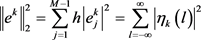,(21)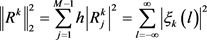. (22)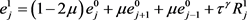,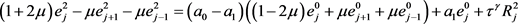,(23)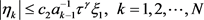.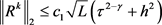(24)

(22)式等式右边的系数数列是收敛的，因此存在一个正数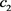使得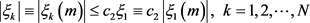. (25)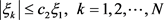.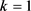时，,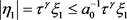,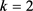时，,,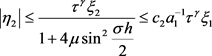,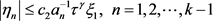...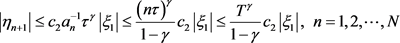.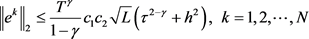.

5. 数值试验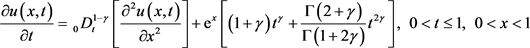.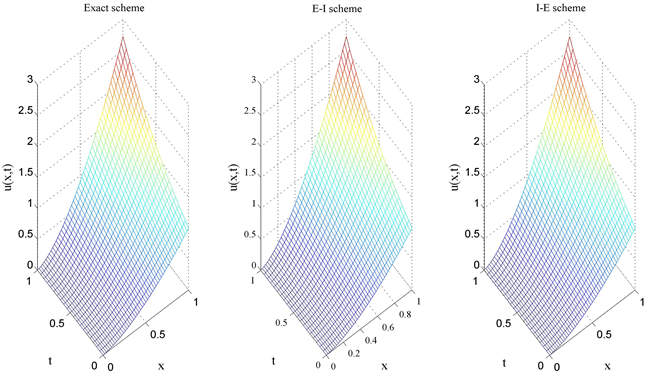Figure 1. Surfaces of analytic solution and E-I, I-E scheme numerical solutions时，给出解析解、显–隐和隐–显格式的解曲面图1；可以看出解析解、显–隐和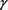分别取0.1~0.9等不同值，取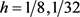，隐式格式、显–隐格式和隐–显格式三种数值格式的最大模误差情况见表1。由表1可知，隐式格式与显–隐和隐–显格式的精度相当；对不同值隐式格式和显–隐格式的误差比较；可以看出当值大于0.5时，隐式格式的精度高于显–隐格式，当值小于0.5时，显–隐格式的精度优于隐式格式。随着值得减小，显–隐格式和隐–显格式的误差越来越小；整数阶显–隐差分格式为二阶差分格式，其精度优于隐式格式。当值接近于0时，显–隐格式和隐–显格式的特性接近整数阶的情形。Table 1. Accuracy comparison analysis of three numerical schemesTable 2. Time direction convergence order of three difference schemes (h = 64)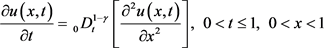.Table 3. Spatial direction convergence order of three difference schemes under different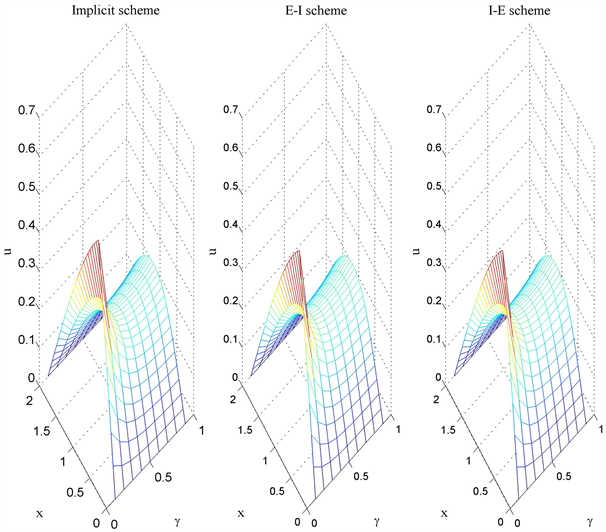Figure 2. Surface of numerical solution of three different schemes for differentTable 4. Comparison of calculation times for three differential schemes (unit: s)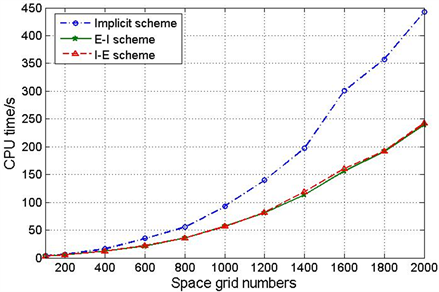Figure 3. Comparison of calculation times for three differential schemes

6. 结论

NOTES

*通讯作者。

  Uchaĭkin, V.V. (2013) Fractional Derivatives for Physicists and Engineers, Volume I: Background and Theory. Springer, Berlin. https://doi.org/10.1007/978-3-642-33911-0  Uchaĭkin, V.V. (2013) Fractional Derivatives for Physicist and Engineers, Volume II: Applications. Springer, Berlin. https://doi.org/10.1007/978-3-642-33911-0  Guo, B.L., Pu, X.K., Huang, F.H., et al. (2015) Fractional Partial Differential Equations and Their Numerical Solutions. Science Press, Beijing. https://doi.org/10.1142/9543  陈文, 孙洪广. 反常扩散的分数阶微分方程和统计模型[M]. 北京: 科学出版社, 2017.  刘发旺, 庄平辉, 刘青霞. 分数阶偏微分方程数值方法及其应用[M]. 北京: 科学出版社, 2015.  孙志忠, 高广花. 分数阶微分方程的有限差分方法[M]. 北京: 科学出版社, 2015.  Li, X.J. and Xu, C.J. (2009) A Space-Time Spectral Method for the Time Fractional Diffusion Equation. Siam Journal on Numerical Analysis, 47, 2108-2131. https://doi.org/10.1137/080718942  Zeng, F.H., Li, C.P., Liu, F.W., et al. (2013) The Use of Finite Difference/Element Approaches for Solving the Time Fractional Sub-Diffusion Equation. SIAM Journal on Scientific Computing, 35, A2976-A3000. https://doi.org/10.1137/130910865  Lv, C.W. and Xu, C.J. (2015) Improved Error Estimates of a Finite Difference/Spectral Method for Time-Fractional Diffusion Equations. International Journal of Numerical Analysis & Modeling, 12, 384-400.  杨莹莹, 李景. 空间分布阶时间分数阶扩散方程的有限体积法[J]. 理论数学, 2019, 9(3): 351-361.  Wang, H. and Wang, K.X. (2011) An O(N log2N) Alternating-Direction Finite Difference Method for Two-Dimensional Fractional Diffusion Equations. Journal of Computational Physics, 230, 7830-7839. https://doi.org/10.1016/j.jcp.2011.07.003  Cui, M.R. (2018) Com-pact Finite Difference Schemes for the Time Fractional Diffusion Equation with Nonlocal Boundary Conditions. Computational & Applied Mathematics, 37, 3906-3926. https://doi.org/10.1007/s40314-017-0553-7  Zhang, Y.N., Sun, Z.Z. and Wu, H.W. (2011) Error Estimates of Crank-Nicolson-Type Difference Schemes for the Sub-Diffusion Equation. SIAM Journal on Numerical Analysis, 49, 2302-2322. https://doi.org/10.1137/100812707  Liu, F.W., Meerschaert, M.M., Mcgough, R.J., et al. (2013) Numerical Methods for Solving the Multi-Term Time-Fractional Wave-Diffusion Equation. Fractional Calculus & Applied Analysis, 16, 9-25. https://doi.org/10.2478/s13540-013-0002-2  Wu, L.F., Yang, X.Z. and Cao, Y.H. (2018) An Alternating Segment Crank-Nicolson Parallel Difference Scheme for the Time Fractional Sub-Diffusion Equation. Advances in Difference Equations, 2018, 287. https://doi.org/10.1186/s13662-018-1749-x  Chen, C.M., Liu, F.W., Turner, I., et al. (2007) A Fourier Method for the Fractional Diffusion Equation Describing Sub-Diffusion. Journal of Computational Physics, 227, 886-897. https://doi.org/10.1016/j.jcp.2007.05.012  Chen, C.M., Liu, F.W. and Anh, V. (2009) A Fourier Method and an Extrapolation Technique for Stokes’ First Problem for a Heated Generalized Second Grade Fluid with Fractional Derivative. Journal of Computational and Applied Mathematics, 223, 777-789. https://doi.org/10.1016/j.cam.2008.03.001  Gao, G.H. and Sun, Z.Z. (2017) Two Difference Schemes for Solving the One-Dimensional Time Distributed-Order Fractional Wave Equations. Numerical Algorithms, 74, 675-697. https://doi.org/10.1007/s11075-016-0167-y  Langlands, T.A.M. and Henry, B.I. (2005) The Accuracy and Stability of an Implicit Solution Method for the Fractional Diffusion Equation. Journal of Computational Physics, 205, 719-736. https://doi.org/10.1016/j.jcp.2004.11.025  孙志忠. 非线性发展方程的有限差分方法[M]. 北京: 科学出版社, 2018.  张锁春. 抛物型方程定解问题的有限差分数值计算[M]. 北京: 科学出版社, 2010.